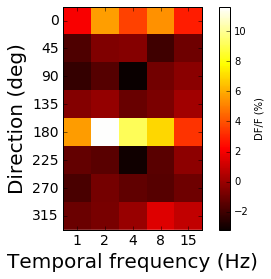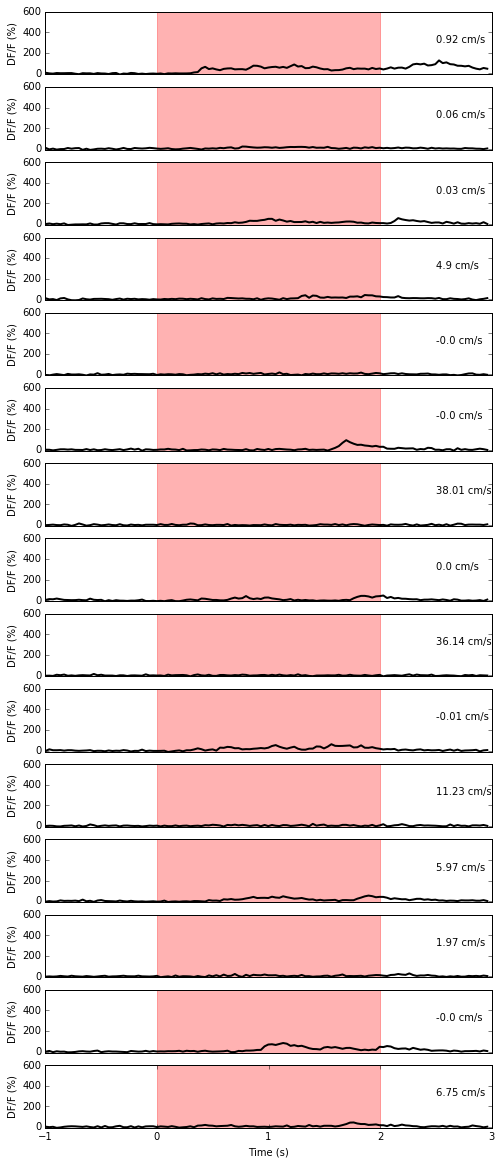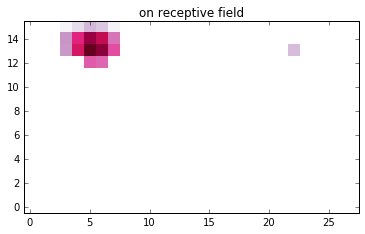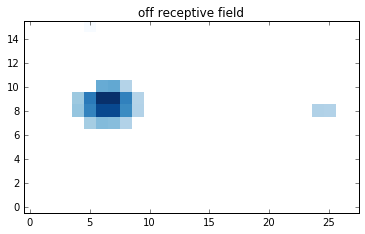## Brain Observatory Trace Analysis¶

This notebook demonstrates how to run the stimulus-specific tuning analysis code in the SDK. First let's instantiate a BrainObservatoryCache instance.

In :
from allensdk.core.brain_observatory_cache import BrainObservatoryCache
boc =  BrainObservatoryCache()


## Drifting Gratings¶

In this example, we'll show how you can plot a heatmap of a cell's response organized by orientation and temporal frequency. Here we start with a known experiment ID. Take a look at the other notebook to see how you can find experiments of interest. You can run the drifting grating analysis code on that experiment's NWB file as follows:

In :
import matplotlib.pyplot as plt
from matplotlib.ticker import MaxNLocator
%matplotlib inline

from allensdk.brain_observatory.drifting_gratings import DriftingGratings

data_set = boc.get_ophys_experiment_data(502376461)
dg = DriftingGratings(data_set)


If you know which cell you're interested in, here's how you can find out where it is in the NWB File.

In :
import numpy as np
specimen_id = 517425074
cell_loc = data_set.get_cell_specimen_indices([specimen_id])

print("Specimen ID:", specimen_id)
print("Cell loc:", cell_loc)

('Specimen ID:', 517425074)
('Cell loc:', 97)


The response property of the stimulus-specific analysis objects is 4-D array organized with the following dimensions:

0: num. grating directions
1: num. grating temporal frequencies + 1 (0=blank sweep)
2: num. cells + 1 (running speed)
3: 0=response mean, 1=response standard error of the mean, 2=number of signficant trials



Dimension 2 of the response array has one index per cell in the experiment, plus one. The final index of that dimension is the running speed (response[:,:,-1,:]). This organization allows users to examine whether the mouse ran more for some specific stimulus conditions.

In :
# skip the blank sweep column of the temporal frequency dimension
plt.imshow(dg.response[:,1:,cell_loc,0], cmap='hot', interpolation='none')
plt.xticks(range(5), dg.tfvals[1:])
plt.yticks(range(8), dg.orivals)
plt.xlabel("Temporal frequency (Hz)", fontsize=20)
plt.ylabel("Direction (deg)", fontsize=20)
plt.tick_params(labelsize=14)
cbar= plt.colorbar()
cbar.set_label("DF/F (%)")The peak property of the analysis object is a Pandas DataFrame of peak conditions (direction and temporal frequency) as well as computed response metrics. For drifting gratings this includes:

ori_dg: preferred direction (index into dg.orivals)
tf_dg: preferred temporal frequency (index into tf.tfvals)
response_reliability_dg: response reliability
osi_dg: orientation selectivity index
dsi_dg: direction selectivity index
ptest_dg: number of signficant cells
p_run_dg: K-S statistic comparing running trials to stationary trials
run_modulation_dg: ratio of mean fluorescence during running vs static
cv_dg: circular variance 
In :
dg.peak.loc[cell_loc]

Out:
ori_dg                         4
tf_dg                          2
reliability_dg          0.194066
osi_dg                   1.21454
dsi_dg                  0.340134
peak_dff_dg              11.6263
ptest_dg             3.03061e-21
p_run_dg               0.0638074
run_modulation_dg        -0.6221
cv_os_dg                       1
cv_ds_dg                0.340134
tf_index_dg             0.297836
cell_specimen_id       517425074
Name: 97, dtype: object

Next let's plot all trials for a given cell's preferred condition.

In :
pref_ori = dg.orivals[dg.peak.ori_dg[cell_loc]]
pref_tf = dg.tfvals[dg.peak.tf_dg[cell_loc]]
print("Preferred direction:", pref_ori)
print("Preferred temporal frequency:", pref_tf)

('Preferred direction:', 180)
('Preferred temporal frequency:', 2)

In :
pref_trials = dg.stim_table[(dg.stim_table.orientation==pref_ori)&(dg.stim_table.temporal_frequency==pref_tf)]
pref_trials

Out:
temporal_frequency orientation blank_sweep start end
1 2 180 0 836 896
71 2 180 0 7156 7216
73 2 180 0 7337 7397
100 2 180 0 9775 9834
141 2 180 0 13477 13536
175 2 180 0 16548 16607
271 2 180 0 55013 55073
291 2 180 0 56819 56878
323 2 180 0 59708 59768
435 2 180 0 97818 97878
449 2 180 0 99082 99142
488 2 180 0 102603 102663
509 2 180 0 104499 104559
518 2 180 0 105312 105371
606 2 180 0 113258 113318

sweep_response is a DataFrame that contains the DF/F of each cell during each stimulus trial. It shares its index with stim_table. Each cell contains a timeseries that extends from 1 second prior to the start of the trial to 1 second after the end of the trial. The final column of sweep_response, named dx, is the running speed of the mouse during each trial. The data in this DataFrame is used to create another DataFrame called mean_sweep_response that contains the mean DF/F during the trial for each cell (and the mean running speed in the last column).

In :
subset = dg.sweep_response[(dg.stim_table.orientation==pref_ori)&(dg.stim_table.temporal_frequency==pref_tf)]


Here we look at the mean running speed during trials that presented the preferred condition.

In :
subset_mean = dg.mean_sweep_response[(dg.stim_table.orientation==pref_ori)&(dg.stim_table.temporal_frequency==pref_tf)]
subset_mean['dx']

Out:
1       0.920868
71      0.060151
73      0.027268
100     4.897258
141    -0.000856
175    -0.002599
271    38.010730
291     0.000437
323    36.139884
435    -0.012866
449    11.234307
488     5.972907
509     1.967057
518    -0.004841
606     6.747042
Name: dx, dtype: float64

Plot the response to each trial of the preferred condition, labeled with the mean running speed during the trial

In :
trial_timestamps = np.arange(-1*dg.interlength, dg.interlength+dg.sweeplength, 1.)/dg.acquisition_rate
plt.figure(figsize=(8,20))
for i in range(len(subset)):
plt.subplot(len(pref_trials),1,i+1)
plt.plot(trial_timestamps, subset[str(cell_loc)].iloc[i], color='k', lw=2)
plt.axvspan(0,2,color='red', alpha=0.3)
plt.ylabel("DF/F (%)")
plt.ylim(-10,600)
plt.yticks(range(0,700,200))
plt.text(2.5, 300, str(round(subset_mean['dx'].iloc[i],2))+" cm/s")
if i<(len(subset)-1):
plt.xticks([])
else:
plt.xticks([-1,0,1,2,3])
plt.xlabel("Time (s)")## Static Gratings¶

The static gratings analysis object is quite similar to the drifting gratings analysis object. Here we'll just take a look at the peak table, which contains information about the preferred orientation, spatial frequency, phase, as well as a number of other metrics.

In :
from allensdk.brain_observatory.static_gratings import StaticGratings

data_set = boc.get_ophys_experiment_data(510938357)

sg = StaticGratings(data_set)

In :
sg.peak.head()

Out:
ori_sg sf_sg phase_sg reliability_sg osi_sg peak_dff_sg ptest_sg time_to_peak_sg cell_specimen_id p_run_sg cv_os_sg run_modulation_sg sf_index_sg
0 4 2 1 0.0113189 0.285308 3.36311 0.559699 0.6634 517399188 0.898808 0.313745 0.193421 0.193012
1 5 3 3 0.0328444 0.856061 17.1593 5.6698e-07 0.46438 517399994 0.102605 0.792459 0.716304 0.272816
2 4 3 3 0.0140488 0.918923 4.73641 0.81548 0.36487 517399857 0.118354 0.602649 0.870513 0.218487
3 5 5 1 -0.00940717 1.05556 2.29522 0.0635452 0.36487 517399727 0.259146 0.914524 1.05157 0.145557
4 3 5 0 -0.00875107 0.378599 3.99094 0.0794371 0.86242 517399442 0.112734 0.318078 0.958211 0.204495

## Natural Scenes¶

The natural scenes analysis object is again similar to the others. In addition to computing the sweep_response and mean_sweep_response arrays, NaturalScenes reports the cell's preferred scene, running modulation, time to peak response, and other metrics.

In :
from allensdk.brain_observatory.natural_scenes import NaturalScenes

data_set = boc.get_ophys_experiment_data(510938357)

ns = NaturalScenes(data_set)
print("done analyzing natural scenes")

done analyzing natural scenes

In :
ns.peak.head()

Out:
scene_ns reliability_ns peak_dff_ns ptest_ns p_run_ns run_modulation_ns time_to_peak_ns cell_specimen_id image_selectivity_ns
0 48 0.0183184 4.91692 0.241369 0.0814777 -1.04747 0.76291 517399188 0.307441
1 96 0.151659 7.58865 2.77309e-08 0.0145825 -1.24205 0.36487 517399994 0.523847
2 103 -0.0106162 2.51431 0.0333866 0.0991697 1.01418 0.3317 517399857 -0.000474576
3 15 0.0047568 1.65234 0.577975 0.156953 1.04387 0.03317 517399727 0.176847
4 21 0.000218728 1.55928 0.0684794 0.428506 0.539678 0.56389 517399442 0.242186

## Locally Sparse Noise¶

The locally sparse noise stimulus object is a bit different from the others. It does not have a peak condition table, instead providing a method to retrieve the "on" and "off" receptive fields of all cells. The receptive field of a cell is computed by averaging responses to trials in which a given sparse noise grid location is on/off.

In :
from allensdk.brain_observatory.locally_sparse_noise import LocallySparseNoise
import allensdk.brain_observatory.stimulus_info as stim_info

specimen_id = 587179530
cell = boc.get_cell_specimens(ids=[specimen_id])

exp = boc.get_ophys_experiments(experiment_container_ids=[cell['experiment_container_id']],
stimuli=[stim_info.LOCALLY_SPARSE_NOISE])

data_set = boc.get_ophys_experiment_data(exp['id'])
lsn = LocallySparseNoise(data_set)
print("done analyzing locally sparse noise")

done analyzing locally sparse noise

In :
import numpy as np
import matplotlib.pyplot as plt
%matplotlib inline

cell_idx = data_set.get_cell_specimen_indices([specimen_id])

plt.imshow(lsn.receptive_field[:,:,cell_idx,0], interpolation='nearest', cmap='PuRd', origin='lower')
plt.title("on receptive field")
plt.show()
plt.imshow(lsn.receptive_field[:,:,cell_idx,1], interpolation='nearest', cmap='Blues', origin='lower')
plt.title("off receptive field")
plt.show()